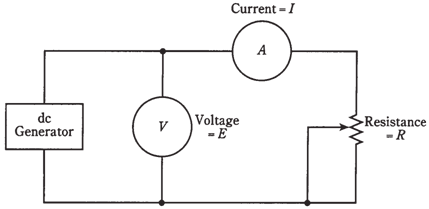## Current calculations Assignment Help

Assignment Help: >> Electrical Engineering - Current calculations

Current calculations

The first way to use Ohm's Law is to find out current values in direct current circuits. To find the current, you should know the voltage and the resistance, or be able to deduce them.

Refer to the schematic diagram of Figure. It consists of a variable direct current generator, some wire, a voltmeter, an ammeter, and a calibrated, wide range potentiometer. Component values have been left out of this diagram, so it is not a drawing a diagram.

But the values are assigned for of creating sample Ohm's Law problems. While calculating the current in the below stated problems, it is essential to mentally "cover up" the meter.Figure--   Circuit for Ohm's Law problems and working.

Problem 1:

Suppose that the direct current generator produces 10 V, and that the potentiometer is set to a value of 10   . Then what will be the current which we obtain?

This is solved by the formula I=E/R. Just plug in values for E and R; they are both 10, as the units were given in volts and ohms. Then I=10/10=1 A.

Problem 2:

The direct current generator produces 100 V and the potentiometer is set to 10 KΩ. What is the current which we obtain?

First, convert the resistance to ohms: 10 KΩ=10,000. And then plug the values in:

I = 100/10,000 = 0.01 A. This can better be expressed as 10 mA.

Engineers and technicians favor to keep the numbers within reason while specifying the quantities. Although it is perfectly all right to say that the current is 0.01 A, it is best if the numbers can be kept at 1 or more, but is certainly less than 1,000. It is a silly to talk about a current of 0.003 A, or a resistance of 107,000 Ω, when you can say 3 mA or 107 KΩ.